# MomentJS - Is Same

This method will check if the moment is same as another moment. It returns true or false.

## Syntax

```moment().isSame(Moment|String|Number|Date|Array);
moment().isSame(Moment|String|Number|Date|Array, String);
```

## Example

```var issame = moment([2015, 10, 01]).isSame([2015, 10, 01]);
```

## Output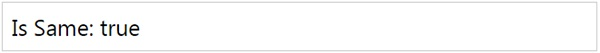Note that as with isBefore, we can use unit with isSame() method. Following are the units supported: year, month, week, day, hour, minute and second.

## Example

```var issame = moment([2015, 10, 01]).isSame([2015, 05, 10], 'year');
```

## Output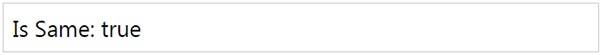Since the year of both moments is matching, it gives true as the output.

## Example

```var issame = moment([2015, 10, 01]).isSame([2015, 05, 10], 'month');
```

## Output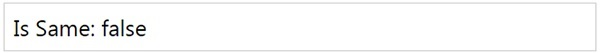Note that in the above example the month is not matching, so it gives the output as false.

You will get false for month unit even if the year is not matching. Observe the following code for the same.

## Example

```var issame = moment([2015, 10, 01]).isSame([2014, 05, 10], 'month');
```

## Output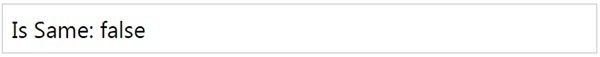## Example

```var issame = moment([2015, 10, 01]).isSame([2015, 05, 10], 'day');
```

## Output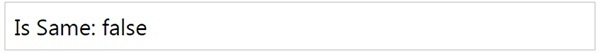momentjs_date_queries.htm